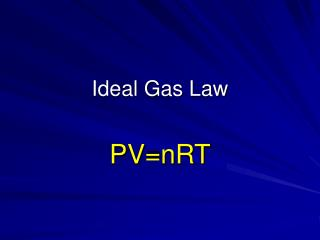DownloadDownload PresentationIdeal Gas Law

# Ideal Gas Law

Télécharger la présentation## Ideal Gas Law

- - - - - - - - - - - - - - - - - - - - - - - - - - - E N D - - - - - - - - - - - - - - - - - - - - - - - - - - -
##### Presentation Transcript

1. Ideal Gas Law PV=nRT

2. Remember… • Boyle’s Law • Charles’ Law: • Combined Gas Law:(Units MUST Match Temp in Kelvin!!!)

3. A gas with a volume of 350 ml is collected at 15o C and 120 kPa. If the temperature changes to 30o C, what pressure would be required to put this gas in a 300 ml container?

4. A balloon has a volume of 500 ml at a temperature of 22oC and a pressure of 755 mmHg. If the balloon is cooled to 0o C and a pressure of 145 mmHg, what is its new volume?

5. Avogadro’s Principle Under similar conditions (same Temp and Pressure) equal volumes of gases contain equal numbers of particles. 10 L of H2 (g) and 10 L of O2 (g)Both at Standard Temperature and Pressure (STP) contain… The same number of particles!

6. Molar Volume The volume of 1 mole of gas particles at STP is 22.4 L

7. Ideal Gas Law Animation:

8. Try this: 1 mole of gas occupies 22.4 L at STP = __________ ml (22400) = ___________ moles of gas (1 mole) = ___________ particles (6.02 x 1023)

9. How many particles in 11.2 dm3 of gas at STP? 0.5 moles = 3.01 x 1023 particles 22,400 cm3 of NH3 gas at STP weighs? = 22.4 L = 1 mole = 17 grams (add up MW) 44.8 L of NH3 at STP weighs? = 2 moles = 34 grams _____ grams = 1 mole of nitrogen gas = _____ L at STP? 28.00 22.4

10. How many N2 molecules are in 22.4 dm3 at STP? = 1 mole = 6.02 x 1023 What volume will 1.2 x 1024 H2 molecules occupy at STP? = 2 moles = 44.8 L at STP

11. Ideal Gas EquationUse when NOT at STP!!! PV= nRT P = Pressure (in kPa) V = Volume (in Liters or dm3) n = number of moles T = Temperature (in Kelvin) R =8.31 L• kPa mole • K

12. Development of R in

13. Finding Molecular Weight of a Gas • Remember: MW = grams / moles • Converting grams to moles • Divide grams by the molecular weight

14. 1) 5.0 L of a gas weighs 30.00 g at 20o C & 92 kPa. What is the mole weight of the gas? PV=nRT 92kPa • 5.0 L= n • 8.31 • 293 K n = 0.19 mol

15. 2)If the mole weight of a gas is 26 g/mol and 18.00 g of the gas is 30 L at 21o C, what is the pressure of the gas? PV= nRT P x 30 L=0.69 mol x 8.31 x 294 K P = 56.2kPa

16. Stoichiometry • Solving Steps. • Balance the Equation • Change grams to moles • Use mole ratio to solve • Change moles to volumes

17. Use MW on P.T. Use MW on P.T. Use mole ratio (coefficients) Use 22.4 L/mol @ STP Or PV = nRT Use 22.4 L/mol @ STP Or PV = nRT

18. Mg (c) + HCl (ag)  MgCl2 + H2 (g) If 2.43 g Mg react what volume of H2 is produced? (at STP) 1:1 0.1 mole 0.1 moles 2 Mg (c) + HCl (ag)  MgCl2 + H2 (g) ? L 2.24 L H2 2.43 g Mg

19. Mg (c) +2 HCl (ag)  MgCl2 + H2 (g) If 2.43 g Mg react what volume of H2 is produced at 40oC and 85 kPa? 1:1 0.1 mole 0.1 moles 2 Mg (c) + HCl (ag)  MgCl2 + H2 (g) ? L 3.06 L H2 2.43 g Mg

20. If 250 ml of H2 is produced at 20o C & 100 kPa, what mass of Mg reacted? 1:1 0.01 mole 0.01 moles 2 Mg (c) + HCl (ag)  MgCl2 + H2 (g) ? g 0.243 g Mg 250 ml

21. Liters B Use mole ratio (coefficients) Volume to Volume THE MOLE RATIO IS THE SAME AS THE VOLUME RATIO. Liters A

22. Burning of methane: What vol. of oxygen is needed to completely burn 1 L of methane? 1:2 1.0 L 2.0 L CH4 + O2 CO2 (g) + H2O (l) 2 2

23. Burning of methane: To produce 11.2 L of CO2 requires how many moles of O2 at STP? 2:1 1 mole = 22.4 L 11.2 L CH4 + O2 CO2 (g) + H2O (l) 2 2